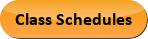Oct 05, 2022
2022-2023 College Catalog
 Select a Catalog 2022-2023 College Catalog 2021-2022 College Catalog [ARCHIVED CATALOG] 2020-2021 College Catalog [ARCHIVED CATALOG] 2019-2020 College Catalog [ARCHIVED CATALOG]
 HELP 2022-2023 College Catalog Print-Friendly Page (opens a new window)

# PHY 210IN - Introductory Mechanics [SUN# PHY 1121]

4 Credits, 6 Contact Hours
3 lecture periods 3 lab periods

Calculus-based introduction to mechanics for physics, engineering, and mathematics majors. Includes nature of physics; linear motion and kinematics; dynamics; work and energy; linear momentum; and rotational motion.

Prerequisite(s): MAT 220
Information: High school physics is strongly recommended before enrolling in this course.
Gen-Ed: Meets AGEC - SCI; Meets CTE - M&S.Course Learning Outcomes
1. Show improvement in the application of physical laws when analyzing natural phenomena and the interaction of physical objects.
2. Demonstrate understanding of force by predicting outcomes in a variety of physical situations.
3. Evaluate graphical models of motion, and apply mathematical models to predict outcomes.
4. Predict outcomes in situations involving work and energy, and apply conservation principles to solve typical real world problems.
5. Predict outcomes in situations involving impulse and momentum, and apply conservation principles to collisions or explosions.

Performance Objectives:
1. Use the meter-kilogram-second-ampere (MKSA) metric system of measurement in lab and in problem solving.
2. Use specific mathematics skills (powers of 10, trigonometric functions, vectors, simultaneous equations, differentiation and integration) to solve physics problems.
3. Identify and solve problems involving constant velocity and constant acceleration.
4. Apply kinematics equations to solve falling body problems.
5. Apply principles of algebra, calculus, and kinematics to solve projectile and circular motion problems.
6. Apply Newton’s three laws of motion and principles of kinematics to solve force (dynamics) problems.
7. Apply concepts of work and energy to solve problems in mechanics.
8. Apply concepts of momentum and impulse (including conservation) to solve collision and separation problems.
9. Apply concepts of rotational kinematics and dynamics to problems involving rotational motion.
10. Apply principles of conservation of energy and angular momentum to solve problems involving rotational motion.
11. Apply Newton’s law of Universal Gravitation to solve problems involving the Earth’s gravitational field and orbital and planetary motion.
12. Apply concepts of simple harmonic motion to a variety of applicable physical phenomena.

Outline:
1. Nature of Physics
1. Science and scientific method
2. Fundamental and derived quantities
2. Linear Motion and Kinematics
1. One dimensional kinematics
1. Constant velocity motion
2. Constant acceleration (kinematic equations – falling bodies)
2. Two dimensional kinematics
1. Vector addition and components
2. Projectile motion
3. Circular motion
3. Dynamics
1. Newton’s laws of motion (force)
1. Gravity – weight
2. Friction
2. Centripetal force
4. Work and Energy
1. Physical definition of work
2. Vector dot product
3. Kinetic and potential energy
4. Conservation of energy, work-energy theorem
5. Linear Momentum
1. Impulse
2. Conservation of linear momentum
1. One dimension
2. Two dimensions
6. Rotational Motion
1. Vector cross product
2. Kinematics
3. Dynamics
4. Angular momentum
5. Rotational work and energy
7. Laws of Universal Gravitational
1. The gravitational field
2. Gravitational potential energy
3. Planetary orbits
8. Simple Harmonic Motion
1. Hooke’s law forces
2. Position, velocity, and acceleration in simple harmonic motion
3. Energy conservation in simple harmonic motion

Effective Term:
Fall 2020